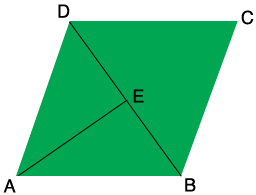SEARCH HOMEMath Central Quandaries & QueriesQuestion from Om, a student: In a rhombus ABCD, angle A=60° and side AB=6 cm. Then diagonal BD is ?Hi Om,What is the measure of the angle ABC? What is the measure of the angle ABD? E is the midpoint of BD. What is the measure of the angle BEA? What trig functions relates the lengths of the sides AB and BE to the measure of the angle ABE?

PennyMath Central is supported by the University of Regina and the Imperial Oil Foundation.